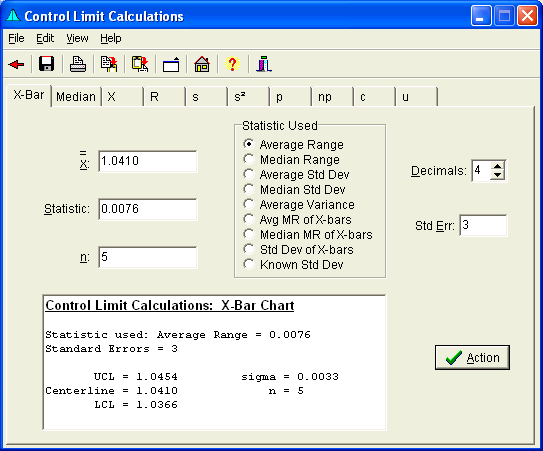# Control Limit Calculations Form

The Control Limit Calculation Form calculates control limits for numerous control charts, each with a number of different calculation methods.

The Variables charts, X-bar, Median, X, R, s, and s2, have a number of different options which can be used to determine the process variation used to determine the control limits. These options include the average and median range, average and median standard deviation, average variance, moving range of the means, standard deviation of the means, or a known standard deviation. Variables charts also provide estimates of the population standard deviation, sigma.

The Attributes control charts have approximate and exact limit calculations as an option. The exact limits for the p and np charts are based on the Binomial Distribution. The exact limits for the c and u charts are based on the Poisson Distribution.

The number of standard errors for each chart may also be modified. The default is 3.